# 基于矩阵分解的协同过滤2019年12月13日07:36:5721 5284字阅读17分36秒

## 简介

• Matrix factorization techniques are usually more effective because they allow us to discover the latent features underlying the interactions between users and items.
• Matrix factorization is simply a mathematical tool for playing around with matrices, and is therefore applicable in many scenarios where one would like to find out something hidden under the data.

## 矩阵分解的协同过滤简单介绍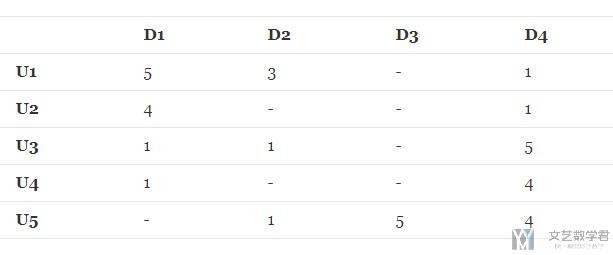## FunkSVD算法

FunkSVD是对于传统SVD的改进, 使其可以用来解决在推荐系统中的问题. 我们下面进行详细的描述.

• 假设我们有U个用户, D个商品.
• 于是我们有了一个U*D大小的矩阵R, 这个矩阵包含所有用户对商品的评价.
• 我们假设我们有K个隐变量 (latent features)
• 我们的任务是, 我们希望可以找到两个矩阵, 矩阵P(U*K大小), 矩阵Q(D*K大小的), 是他们的乘积与原始矩阵R接近. 计算式子如下图所示: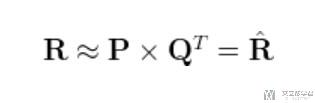• 矩阵P的每一行代表某个人对于不同特征的热爱程度.
• 矩阵Q的每一行代表某个事物拥有这个特征的程度.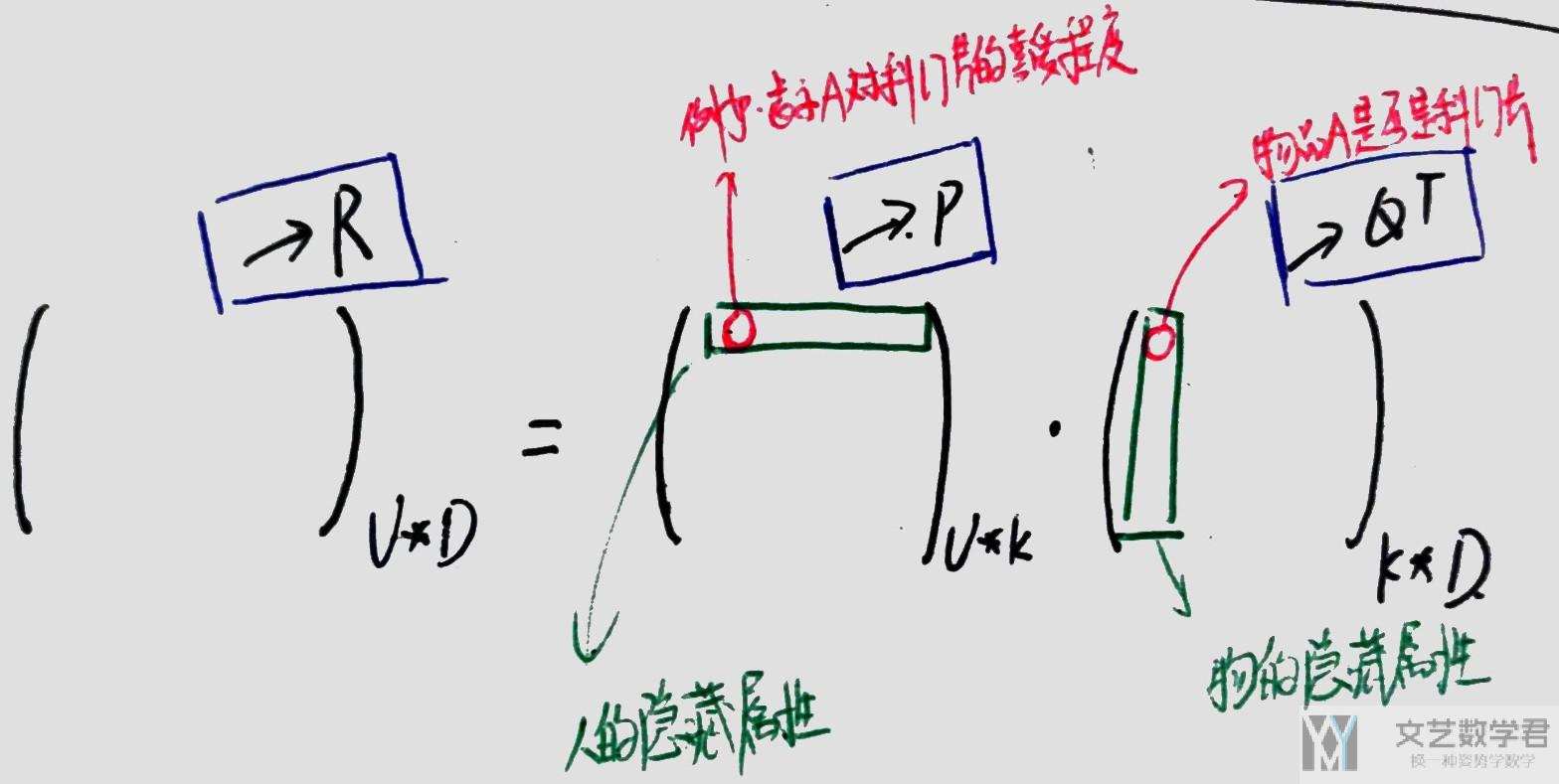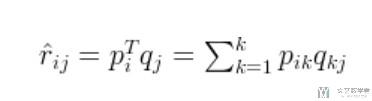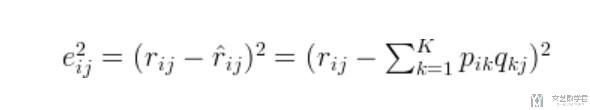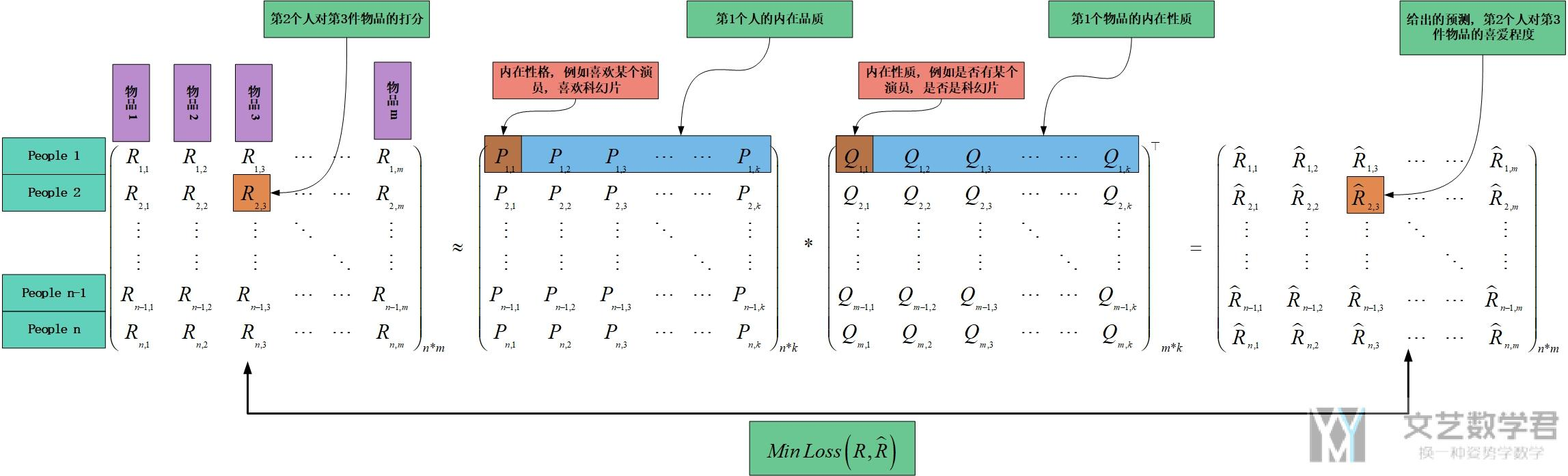## FunkSVD+Regularization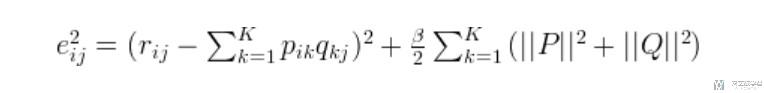### 代码实现

1. import numpy as np
2. import pandas as pd
3. import os
4. import matplotlib.pyplot as plt
5. from datetime import date,datetime
6. import logging
7. import torch
8. from torch import nn
9. from torch import optim
11. device = torch.device("cuda" if torch.cuda.is_available() else "cpu")
12. device
13. # --------------------
14. # 下面三种计算loss的方式
15. # --------------------
16. def PredictR(P, Q, R):
17.     """
18.     最基本的FunkSVD
19.     """
20.     R_pred = torch.mm(P, Q.t()) # 矩阵相乘
21.     N,M = R.shape
22.     loss = 0
23.     for i in range(N):
24.         for j in range(M):
25.             if R[i][j] > 0:
26.                 loss = loss + (R_pred[i][j]-R[i][j])**2
27.     return loss
28. def PredictRegularizationR(P, Q, R):
29.     """
30.     FunkSVD+Regularization
31.     """
32.     B = 0.02 # 正则话的系数
33.     R_pred = torch.mm(P, Q.t()) # 矩阵相乘
34.     N,M = R.shape
35.     loss = 0
36.     for i in range(N):
37.         for j in range(M):
38.             if R[i][j] > 0:
39.                 loss = loss + (R_pred[i][j]-R[i][j])**2
40.     loss = loss + B*((P**2).sum() + (Q**2).sum()) # 加上正则项
41.     return loss
42. def PredictRegularizationConstrainR(P, Q, R):
43.     """
44.     FunkSVD+Regularization+矩阵R的约束(取值只能是0-5, P,Q>0)
45.     """
46.     B = 0.1 # 正则话的系数
47.     R_pred = torch.mm(P, Q.t()) # 矩阵相乘
48.     N,M = R.shape
49.     loss = 0
50.     for i in range(N):
51.         for j in range(M):
52.             if R[i][j] > 0:
53.                 loss = loss + (R_pred[i][j]-R[i][j])**2
54.             elif R[i][j] == 0: # 下面是限定R的范围
55.                 if R_pred[i][j] > 5:
56.                     loss = loss + (R_pred[i][j]-5)**2
57.                 elif R_pred[i][j] < 0:
58.                     loss = loss + (R_pred[i][j]-0)**2
59.     loss = loss + B*((P**2).sum() + (Q**2).sum()) # 加上正则项
60.     # 限定P和Q的范围
61.     loss = loss + ((P[P<0]**2).sum() + (Q[Q<0]**2).sum())
62.     return loss
63. if __name__ == "__main__":
64.     # 原始矩阵R
65.     R = np.array([[5.0, 3.0, 0.0, 1.0],
66.     [4.0, 0.0 ,0.0 ,1.0],
67.     [1.0, 1.0, 0.0, 5.0],
68.     [1.0, 0.0, 0.0, 4.0],
69.     [0.0, 1.0, 5.0, 4.0]])
70.     N,M = R.shape
71.     K = 2
72.     R = torch.from_numpy(R).float()
73.     # 初始化矩阵P和Q
74.     P = Variable(torch.randn(N, K), requires_grad=True)
75.     Q = Variable(torch.randn(M, K), requires_grad=True)
76.     # -----------
77.     # 定义优化器
78.     # -----------
79.     learning_rate = 0.02
81.     lr_scheduler = torch.optim.lr_scheduler.StepLR(optimizer, step_size=1000, gamma=0.9) # 学习率每20轮变为原来的90%
82.     # ---------
83.     # 开始训练
84.     # ---------
85.     num_epochs = 5000
86.     for epoch in range(num_epochs):
87.         lr_scheduler.step()
88.         # 计算Loss
89.         loss = PredictRegularizationConstrainR(P, Q, R)
90.         # 反向传播, 优化矩阵P和Q
92.         loss.backward() # 反向传播
93.         optimizer.step() # 进行优化
94.         if epoch % 20 ==0:
95.             print(epoch,loss)
96.     # 求出最终的矩阵P和Q, 与P*Q
97.     R_pred = torch.mm(P, Q.t())
98.     print(R_pred)
99.     print('-'*10)
100.     print(P)
101.     print('-'*10)
102.     print(Q)

• PredictR: 基本的FunkSVD的思想
• PredictRegularizationR: 基本的FunkSVD+正则化
• PredictRegularizationConstrainR: FunkSVD+正则化+限制R, P, Q的范围, 其中我们希望R的范围在0-5之间, P和Q都可以大于0.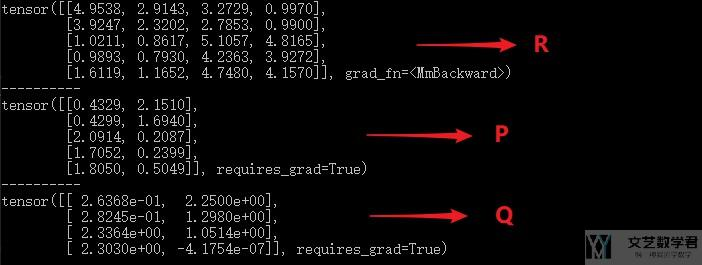## BiasSVD算法

• 用户偏置 : 可以看作用户的性格 , 个人的品质等因素
• 物品偏置 : 例如物品的价格 , 物品的质量等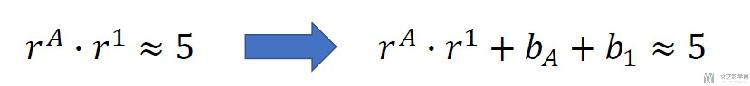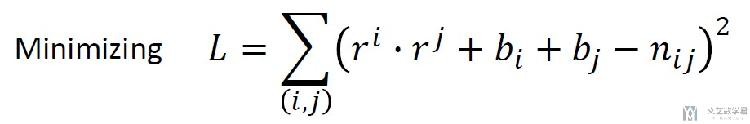• 微信公众号
• 关注微信公众号
•• QQ群
• 我们的QQ群号
•• 本文由 发表于 2019年12月13日07:36:57
• 转载请务必保留本文链接：https://mathpretty.com/11495.html

•CT0011111123S

PyTorch有一些问题而且性能比较慢，建议看https://blog.fastforwardlabs.com/2018/04/10/pytorch-for-recommenders-101.html

•王 茂南

我大致看了一下, 他文章最后好像没说Pytorch的一些问题, 他原文是, “We thought PyTorch was fun to use; models can be built and swapped out relatively easily. When we did encounter errors, most of them were triggered by incorrect data types.” (不过这一篇写的非常不错)